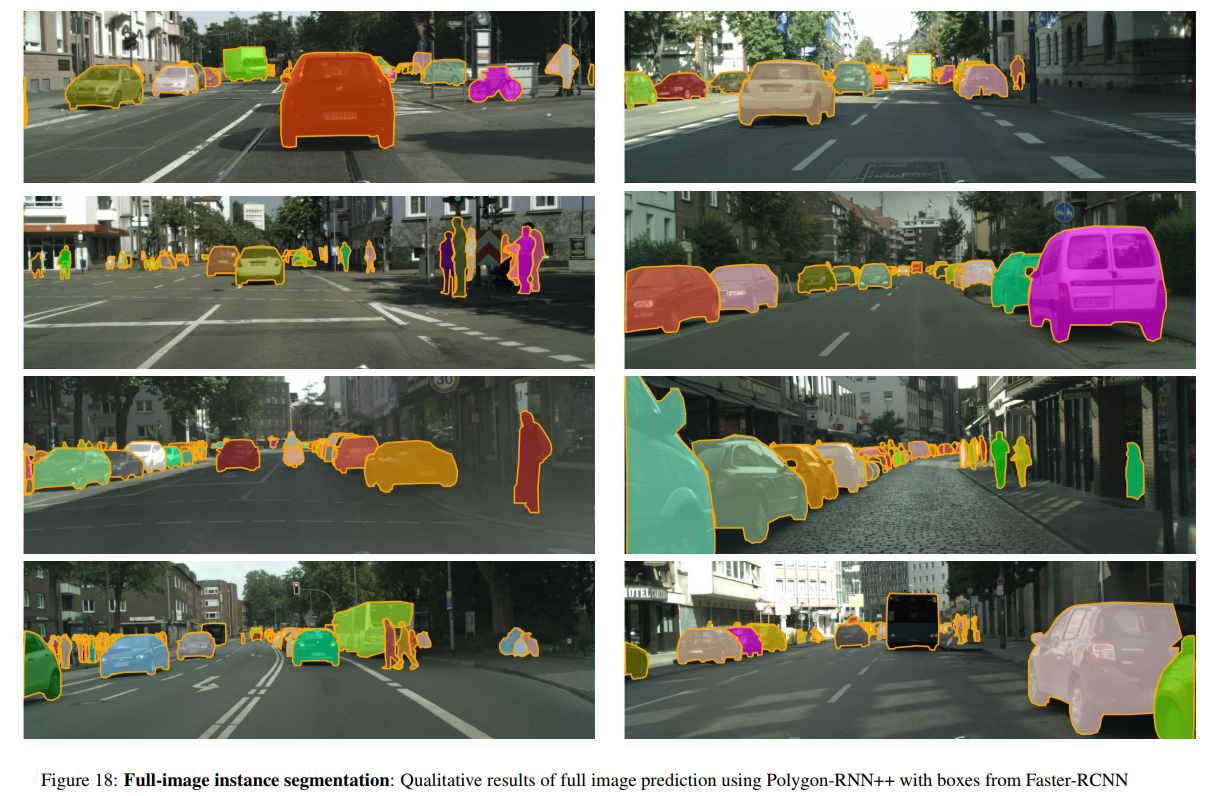## Summary

Everything is there : CNN, RNN, Reinforcement Learning, Gated Graph Neural Network!

Automatic/interactive instance segmentation by building a polygon around the object from a provided bounding box (NOTE: Bounding boxes are assumed to be provided. They can be either human GT or generated by Faster-RCNN.)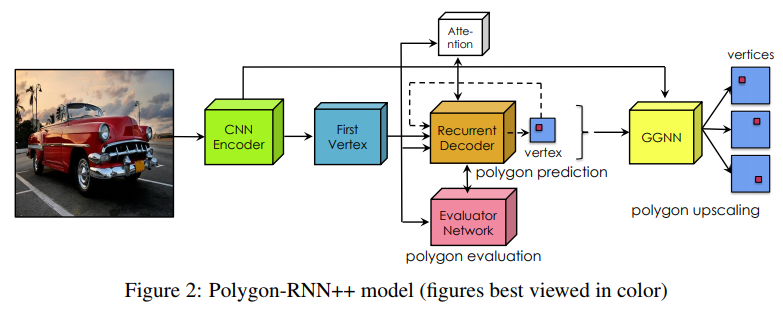1) CNN predicts the first vertex, and provides features for the GGNN

2) RNN predicts multiple sequences of vertices (beam search) to build rough (28x28) candidate polygons

3) “Evaluator” network scores polygons to keep the best

4) GGNN upscales and readjusts polygons

Semi-supervised: Annotator can correct vertices in sequential order, and the model then re-predicts the rest of the polygon

### CNN

• Modified ResNet-50: dilations, reduced strides and skip connections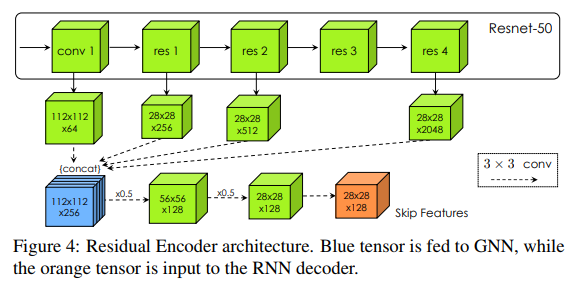### RNN

• 2-layer convolutional LSTM + attention
• Prediction: [(28 x 28) + 1] one-hot vector (last bit is used for end-of-sequence token)

### Reinforcement Learning

• Initialize with cross-entropy, then use RL
• Train using IoU instead of cross-entropy loss
• Reduce exposure bias problem (teacher forcing)
• REINFORCE, with 1 sample Monte Carlo + greedy baseline

### Evaluator Network

• Small ConvNet predicts IoU
• Input: CNN features + last ConvLSTM state + polygon
• Structure: 3x3 conv -> 3x3 conv -> FC
• Trained separately after RL fine-tuning
• L2 loss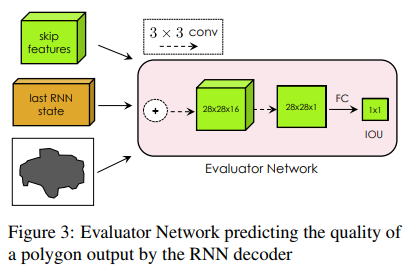### Gated Graph Neural Network

• Extends RNN to graphs using message passing between nodes for a predefined number of steps (T=5)
• Input: polygon represented as a cyclic graph; for each node, a patch is extracted from the image
• Prediction : relative displacement in a D’xD’ grid (treated as a classification task)
• Cross-entropy loss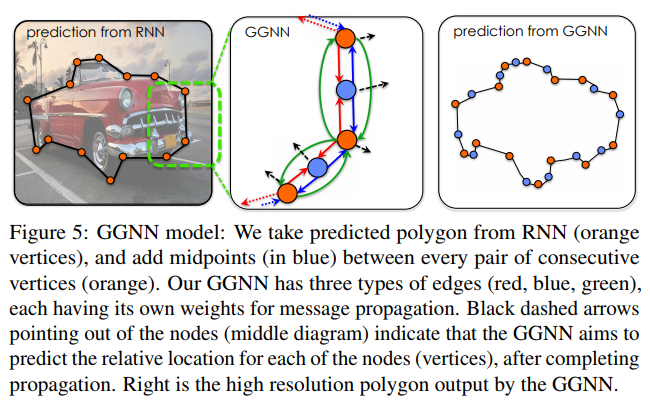## Results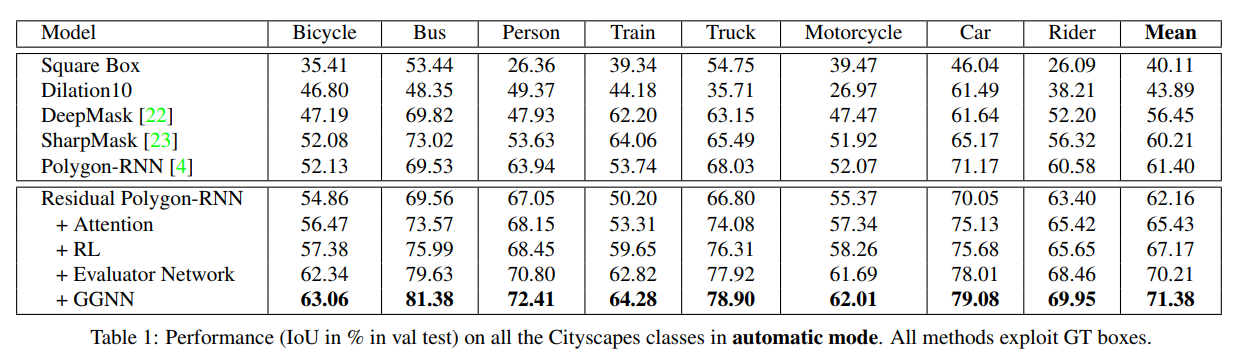### Cityscapes automatic segmentation (GT vs Faster-RCNN boxes)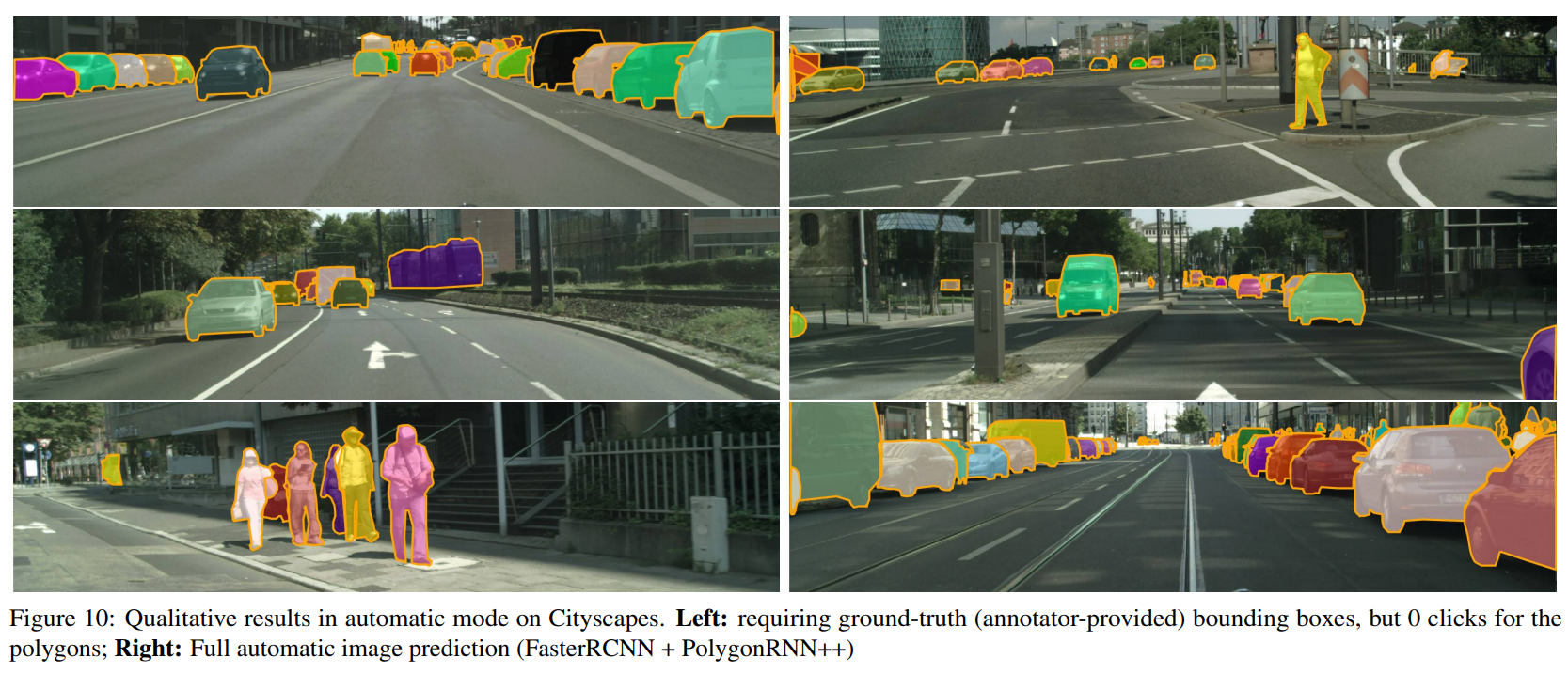### Cityscapes automatic segmentation vs human annotator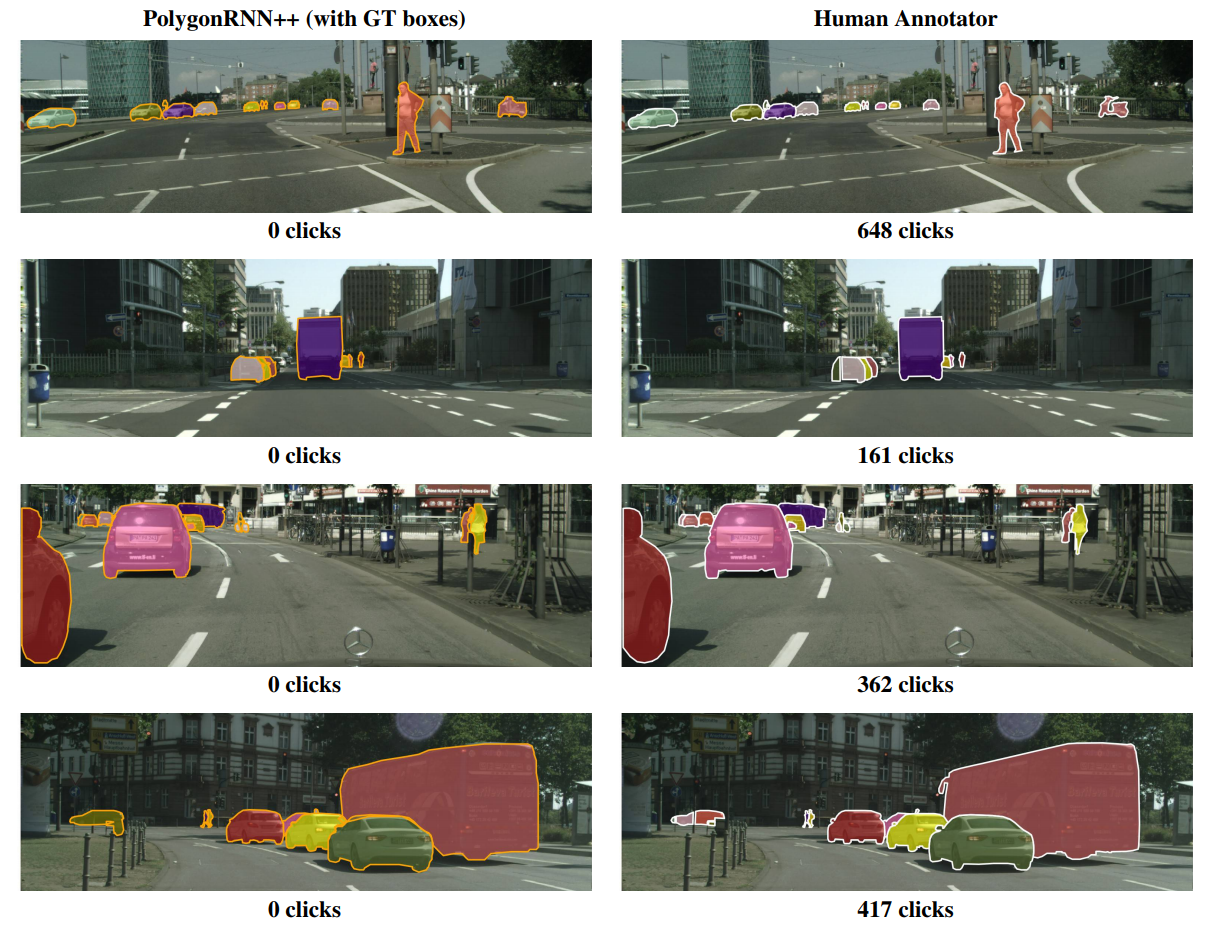### Faster-RCNN + Polygon-RNN++